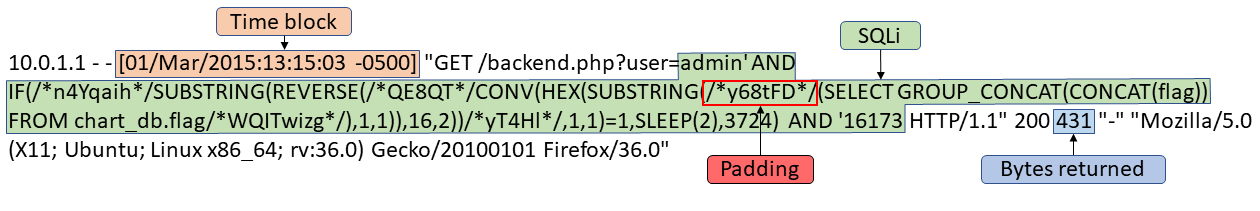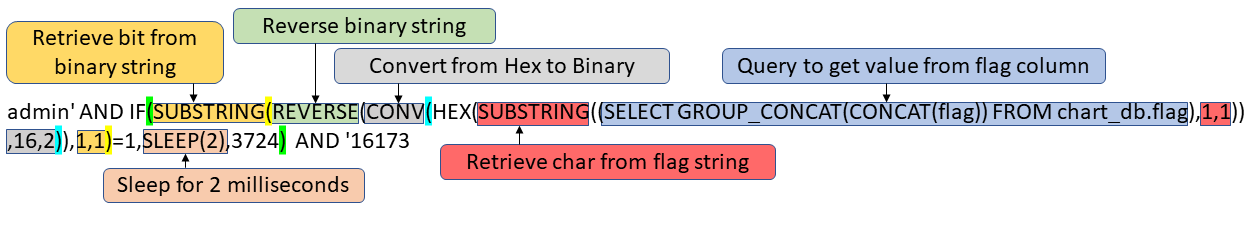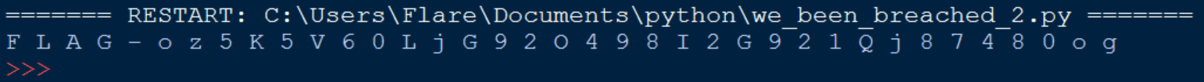# We Got Breached Again from RingZer0team.com

This is an attack similar to the We Got Breached challenge, except this time we only get the activity logs. This challenge can be solved 2 ways, using the time difference between queries or through number of bytes returned.

When the SQLi query is broken out, you can see that the attacker built the query to check each bit of each char. Unlike the previous attack there is no guessing, but you have to make 7 queries for each char which can be noisy. If the bit being checked is 1, then the server sleeps for 2 milliseconds.Anything between “/*” and “*/” is padding used to make the query harder to read, and the number of bytes returned to be different. The same with the random numbers, both in the false branch of the if statement and the last number. This makes it harder to see that the bytes returned can be used to figure out if the if statement returned true or false.If SLEEP(2) executes, then the query is admin’ AND 0 AND ‘16173 = false Else the query is admin’ AND 3724 AND ‘16173 = true

To reconstruct the char bit by bit, the time needs to be compared with the time of the next attack. I first grep for “user=admin’ AND”, since this can only be found in the SQLi queries. Like the previous attack the attacker mapped out the tables to find what was interesting. At 01/Mar/2015:13:15:03 the attacker starts querying the value the flag column. To limit the logs for just the queries referencing the flag column, you can grep for “flag”. A python script can then be put together to automate each check.

``````filepath = 'C:\Users/Flare/Desktop/access_log_flag_only.txt'

with open(filepath) as fp:
cur_bin = ''
cur_time = '13:15:03'
bin_pos = 0

for line in fp:
line_time = line[26:34]

if line_time == cur_time:
cur_bin += '0'
else:
cur_bin += '1'
cur_time = line_time
bin_pos += 1

if len(cur_bin) == 7:
print chr(int(cur_bin[::-1], 2)),
cur_bin = ''
bin_pos = 0
``````

You can also find the flag by checking the number of bytes returned. Since it is the same page as the previous attack the information returned should be the same. The data returned was “query + was/was not found”. This means that more bytes should be returned if the query is false. To get the base line of bytes not due to the query we can do [bytes returned] - len([query]). The number of bytes not due to the query are 229, and 233. The 233 bytes corresponds to the query being false, or not found. Mysql sleep() returns 0 after it has been executed, this means if the if statement is true the query will be false. Below is the python script to automate checking.

``````filepath = 'C:\Users/Flare/Desktop/access_log_flag_only.txt'

with open(filepath) as fp:
bin_pos = 0
cur_char = ''

for line in fp:
start = line.find('user=') + 5
end = line.find('HTTP') - 1
packet_len_pos = line.find(' 200 ') + 5
packet_len = int(line[packet_len_pos:packet_len_pos + 3])

if packet_len - (end - start) == 229:
cur_char += '0'
else:
cur_char += '1'
bin_pos += 1

if bin_pos == 7:
bin_pos = 0
print chr(int(cur_char[::-1], 2)),
cur_char = ''
``````Written on January 8, 2018